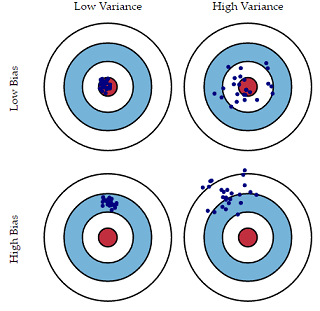This tutorial explains the concept of bias-variance tradeoff in machine learning. It is one of the most commonly confused term in predictive modeling world.

Bias

It refers to model fitting the training data poorly but able to produce similar result in data outside training data. It is related to underfitting. In simple/layman's term, we are building simple models that predicts terribly far from the reality but they don't change much from dataset to dataset.

For example, a linear regression model would have high bias when trying to model a non-linear relationship. It is because linear regression model does not fit non-linear relationship well.

1. High bias means linear regression applied to quadratic relationship.
2. Low bias means second degree polynomial applied to quadratic data.

High Bias Techniques
Linear Regression, Linear Discriminant Analysis and Logistic Regression
Low Bias Techniques
Decision Trees,  K-nearest neighbours and Gradient Boosting

We can also interpret like this -
Parametric algorithms which assume something about the distribution of the data points suffer from High Bias. Whereas non-parametric algorithms which does not assume anything special about distribution have low bias.

Variance

We are building complex models that fits well on training data but they cannot generalise the pattern well which results to overfitting. It means they don't fit well on data outside training (i.e. validation / test datasets). In simple terms, it means they might predict close to reality on average, but they tend to change much more with small changes in the input.

An algorithm like Decision Tree has low bias but high variance, because it can easily change as small change in input variable. In general, it does not generalize the pattern well. It leads to overfitting.

Low Variance Techniques
Linear Regression, Linear Discriminant Analysis, Random Forest, Logistic Regression
High Variance Techniques
Decision Trees,  K-nearest neighbours and Support Vector Machine (SVM)

It means there is a trade-off between predictive accuracy and generalization of pattern outside training data. Increasing the accuracy of the model will lead to less generalization of pattern outside training data. Increasing the bias will decrease the variance. Increasing the variance will decrease the bias.Bias Variance Tradeoff
In the image above, the red ball is the target. Any hit close to it is considered as low bias data points. If each subsequent hit is close to the previous hit is considered as low variance cases.

How to correct Bias-Variance Error

1. Try smaller number of predictors (only important ones) when you have high variance

2. Try larger number of predictors or transform predictors when you have high bias

3. Get more training data when you have high variance

Practical Approaches

1. In support vector machine (SVM), cost (c) parameter decides bias-variance. A large C gives you low bias and high variance. Low bias because you penalize the cost of misclassification a lot. Large C makes the cost of misclassification high, thus forcing the algorithm to explain the input data stricter and potentially overfit. A small C gives you higher bias and lower variance. Small C makes the cost of misclassification low, thus allowing more of them for the sake of wider "cushion"

2. In k-nearest neighbors algorithm, trade-off can be changed by increasing the value of k which increases the number of neighbors that contribute to the prediction and in turn increases the bias of the model and low variance.

3. In decision trees, pruning of tree is a method to reduce variance. It reduces the size of decision trees by removing sections of the tree that provide little power to classify instances.

Training and Cross-Validation Error
1. High Variance - High difference between cross-validation error and the training set error. To overcome it, use more training data. If it is not possible to include more data, include only important predictors or independent variables.
2. High Bias - Low difference between cross-validation and training error. Try more predictors or transform existing predictors.Share
Related PostsDeepanshu founded ListenData with a simple objective - Make analytics easy to understand and follow. He has over 10 years of experience in data science. During his tenure, he has worked with global clients in various domains like Banking, Insurance, Private Equity, Telecom and Human Resource.

3 Responses to "Understanding Bias-Variance Tradeoff"
1.Thank you for educating with such a nice and clean article. It is very easy to understand. Once gain thnq

2.Complex terminologies in simple terms. Great article!

3.hi Deepanshu,
Great concept.Can you also provide R codes to get the bias and variance tradeoff value and how do I know that model is overfitted/underfitted in R

Regards,
Soumita

Next → ← Prev